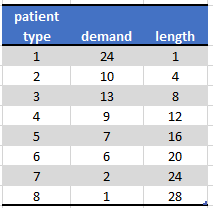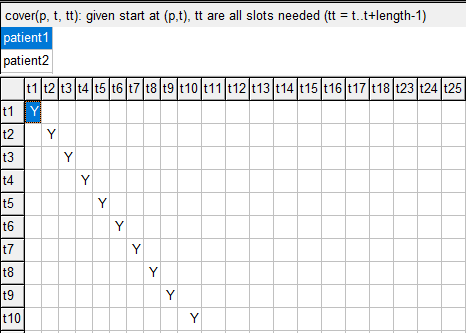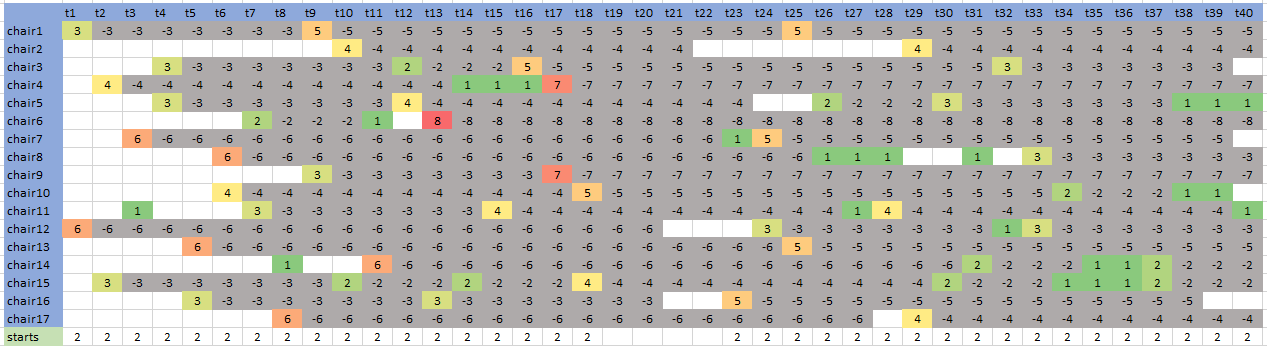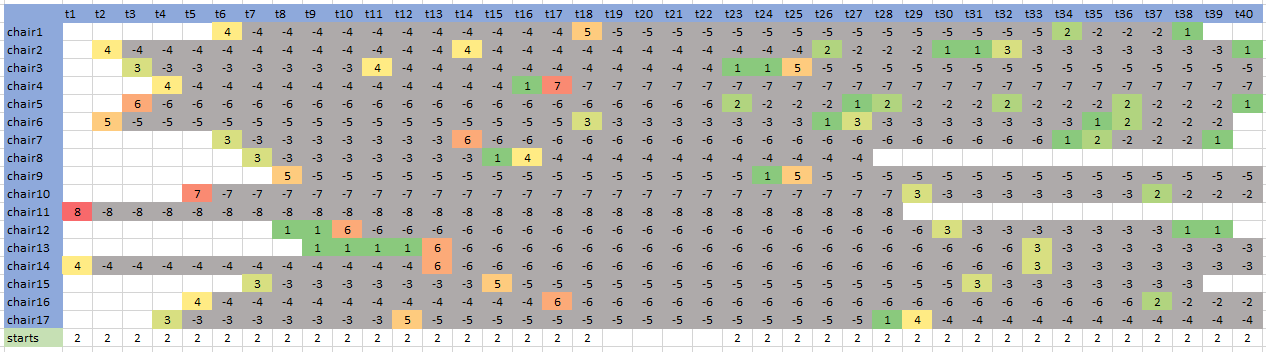## Friday, July 27, 2018

### Scheduling of patients

In  a simple optimization model is presented for the scheduling of patients receiving cancer treatments.  tried to use this model. This was not so easy: small bugs in  can make life difficult when replicating things.

We use the data set in :

• There are $$T=40$$ time slots of 15 minutes
• We have 23 chairs where patients receive their treatment
• We have 8 different types of patients
• Each patient type has a demand (number of actual patients) and a treatment length (expressed in 15 minute slots)
• There is a lunch break during which no patients can start their treatment
• We want at most 2 treatment sessions starting in each time slot.Patient Data

#### Main variables

A treatment session is encoded by two binary variables: $\mathit{start}_{c,p,t} = \begin{cases} 1 & \text{if session for a patient of type p starts in time slot t in infusion chair c} \\ 0 & \text{otherwise} \end{cases}$ $\mathit{next}_{c,p,t} = \begin{cases} 1 & \text{if session for a patient of type p continues in time slot t in infusion chair c} \\ 0 & \text{otherwise} \end{cases}$Start and Next variables
Here the start variables are colored orange and the next variables are grey. Patient type 1 has a treatment session length of 1. This means a session has a start variable turned on, but no next variables.  Patient type 2 has a length of 4. So each session has one start variable and 3 next variables with values one.

Note that there are multiple patients of type 1 and 2.

#### Equations

A chair can be occupied by zero or one patients: $\sum_p \left( \mathit{start}_{c,p,t} + \mathit{next}_{c,p,t}\right)\le 1 \>\forall c,t$

When $$\mathit{start}_{c,p,t}=1$$ we need that the next $$\mathit{length}(p)-1$$ slots have $$\mathit{next}_{c,p,t'}=1$$. Here the paper  makes a mistake. They propose to model this as: $\sum_{t'=t+1}^{t+\mathit{length}(p)-1} \mathit{next}_{c,p,t'} = (\mathit{length}(p)-1)\mathit{start}_{c,p,t}\>\>\forall c,p,t$ This is not correct: this version would imply that we have $\mathit{start}_{c,p,t}=0 \Rightarrow \sum_{t'=t+1}^{t+\mathit{length}(p)-1} \mathit{next}_{c,p,t'}= 0$ This would make a lot of slots just unavailable. (Your model will most likely be infeasible). The correct constraint is:$\sum_{t'=t+1}^{t+\mathit{length}(p)-1} \mathit{next}_{c,p,t'} \ge (\mathit{length}(p)-1)\mathit{start}_{c,p,t}\>\>\forall c,p,t$ A dis-aggregated version is: $\mathit{next}_{c,p,t'} \ge \mathit{start}_{c,p,t} \>\>\forall c,p,t,t'=t+1,\dots\,t+\mathit{length}(p)-1$ This may perform a little bit better in practice (although some solvers can do such a dis-aggregation automatically).

It is noted with this formulation, we only do $\mathit{start}_{c,p,t}=1 \Rightarrow \mathit{next}_{c,p,t'}=1$ If $$\mathit{start}_{c,p,t}=0$$, we leave $$\mathit{next}_{c,p,t'}$$ unrestricted. This mean we have some $$\mathit{next}$$ variables just floating. They can be zero or one. Only the important cases are bound to be one. This again means that the final solution is just $$\mathit{start}_{c,p,t}$$, and we need to reconstruct the $$\mathit{next}$$ variables afterwards. This concept of having variables just floating in case they do not matter, can be encountered in other MIP models.

To meet demand we can do:$\sum_{c,t} \mathit{start}_{c,p,t} = \mathit{demand}_p$

Finally, lunch is easily handled by fixing $\mathit{start}_{c,p,t}=0$ when $$t$$ is part of the lunch period.

There is one additional issue: we cannot start a session if there are not enough time slots left to finish the session. I.e. we have: $\mathit{start}_{c,p,t}=0\>\>\text{if t\ge T - \mathit{length}(p)+2}$

#### GAMS model

The data looks like:

 set   c 'chair' /chair1*chair23/   p 'patient type' /patient1*patient8/   t 'time slots' /t1*t40/   lunch(t) 'lunch time' /t19*t22/ ; alias(t,tt); table patient_data(p,*)             demand  length  patient1     24     1  patient2     10     4  patient3     13     8  patient4      9    12  patient5      7    16  patient6      6    20  patient7      2    24  patient8      1    28 ; scalar maxstart 'max starts in period' /2/ ; parameter    demand(p)    length(p) ; demand(p) = patient_data(p,'demand'); length(p) = patient_data(p,'length');

We create some sets to help us make the equations simpler. This is often a good idea: sets are easier to debug than constraints. Constraints can only be verified when the whole model is finished and we can solve it. Sets can be debugged in advance. In general, I prefer constraints to be as simple as possible.

 set   startok(p,t)  'allowed slots for start'   after(p,t,tt) 'given start at (p,t), tt are further slots needed (tt = t+1..t+length-1)' ; startok(p,t) = ord(t)<=card(t)-length(p)+1; startok(p,lunch) = no; after(p,t,tt) = startok(p,t) and (ord(tt)>=ord(t)+1) and (ord(tt)<=ord(t)+length(p)-1);

The set startok looks likeSet startok
Note that lunch time slots (periods 19-22) are not available.

The set after is a bit more complicated:set after
We see here for patient type 5, given what the start period is (the start period is on the left), when the next variable needs to be turned on. E.g. when patient type 5 starts a treatment session in period 1, the next variables need to be one for periods 2 through 16. At the bottom we see again the effect of the lunch period.

The optimization model can now be expressed as:

 binary variables   start(c,p,t) 'start: begin treatment'   next(c,p,t) 'continue treatment' ; variable z 'objective variable'; start.fx(c,p,t)$(not startok(p,t)) = 0; equations obj 'dummy objective: find feasible solution only' slots(c,p,t) 'start=1 => corresponding next = 0,1' slots2(c,p,t,tt) 'disaggregated version' chair(c,t) 'occupy once' patient(p) 'demand equation' starts(t) 'limit starts in each slot' ; * dummy objective obj.. z =e= 0; * aggregated version slots(c,startok(p,t)).. sum(after(p,t,tt), next(c,p,tt)) =g= (length(p)-1) * start(c,p,t); * disaggregated version slots2(c,after(p,t,tt)).. next(c,p,tt) =g= start(c,p,t); * occupation of chair chair(c,t).. sum(p, start(c,p,t) + next(c,p,t)) =l= 1; * demand equation patient(p).. sum((c,t),start(c,p,t)) =e= demand(p); * limit starts starts(t).. sum((c,p),start(c,p,t)) =l= maxstart; model m1 /slots,chair,patient,starts,obj/; model m2 /slots2,chair,patient,starts,obj/; solve m1 minimizing z using mip; display start.l; I try to make the results more meaningful:  parameter results(*,t) 'reporting'; start.l(c,p,t) = round(start.l(c,p,t)); loop((c,p,t)$(start.l(c,p,t)=1),  results(c,t) = ord(p);  results(c,tt)\$after(p,t,tt) = -ord(p); ); results('starts',t) = sum((c,p),start.l(c,p,t));

We only use the start variables. We know that some of the next variables may have a value of one while not being part of the schedule. The results look like:Results

The colored cells with positive numbers correspond to a start of a session. The grey cells are patients occupying a chair for the remaining time after the start slot. We see that each period has two starts, except for lunch time, when no new patients are scheduled.

This model solves very quickly: about half a second.

#### Proper Next variables

In this model we only use the start variables for reporting. The next variables can show spurious values $$\mathit{next}_{c,p,t}=1$$ which are not binding. Can we change the model so we only have valid next variables?

There are two ways:

1. Minimize the sum of next variables: $\min \sum_{c,p,t} \mathit{next}_{c,p,t}$ Surprisingly this made the model much more difficult to solve.
2. We know in advance how many next variables should be turned on. So we can add the constraint:$\sum_{c,p,t} \mathit{next}_{c,p,t} = \sum_p \mathit{demand}_p (\mathit{length}(p)-1)$ This will prevent these floating next variables.

#### A better formulation

We can remove the next variables altogether and use the start variables directly in the constraint that checks the occupation of chairs: $\sum_p \sum_{t'=t-\mathit{length}_p+1}^t \mathit{start}_{c,p,t'} \le 1 \> \forall c,t$ In GAMS we can model this as follows. We just need to change the set after a little bit: we let tt in after(p,t,tt) include t itself. Let's call this set cover:

 set   startok(p,t)  'allowed slots for start'   cover(p,t,tt) 'given start at (p,t), tt are all slots needed (tt = t..t+length-1)' ; startok(p,t) = ord(t)<=card(t)-length(p)+1; startok(p,lunch) = no; cover(p,t,tt) = startok(p,t) and (ord(tt)>=ord(t)) and (ord(tt)<=ord(t)+length(p)-1);

Note again that the only difference with our earlier set after(p,t,tt) is that after has (ord(tt)>=ord(t)+1)  while cover(p,t,tt) has a condition: (ord(tt)>=ord(t)). One obvious difference between the sets after and cover is the handling of patient type 1. After did not have entries for this patient type, while cover shows:Set cover has a diagonal structure for patient type 1

With this new set cover we can easily form our updated constraint chair:

 * occupation of chair chair(c,t)..   sum(cover(p,tt,t), start(c,p,tt)) =l= 1;

This will find all variables start(c,p,tt) that potentially cover the slot (c,t). Here we see how we can simplify equations a lot by using well-designed intermediate sets.

#### Minimize number of chairs

We can tighten up the schedule a little bit. There is enough slack in the schedule that we actually don't need all chairs to accommodate all patients. To find the minimum number of chairs we make the following changes to the model: first we introduce a new binary variable usechair(c). Next we change the equations:

 * objective obj..  z =e= sum(c, usechair(c)); * occupation of chair chair(c,t)..   sum(cover(p,tt,t), start(c,p,tt)) =l= usechair(c); * chair ordering order(c-1).. usechair(c) =l= usechair(c-1);

The last constraint says $$\mathit{usechair}_c \le \mathit{usechair}_{c-1}$$ for $$c\gt 1$$ (this last condition is implemented by indexing the constraint as order(c-1)). The purpose of this constraint is two-fold: (1) reduce symmetry in the model which hopefully will speed up things, and (2) make the solution better looking: all the unused chairs are at the end. With this, an optimal schedule looks like:Minimize number of chairs

#### Multi-objective version

We can try to make the schedule more compact: try to get rid of empty chairs in the middle of the schedule. An example of such a "hole" is cell: (chair6, t12). We do this by essentially solving a bi-objective problem:
1. Minimize number of chairs needed
Here the spread of a single chair is defined by last slot minus first slot the chair is used. The total spread is just the sum of these. We want to make the minimization of the number of chairs the first, most important objective. The bi-objective problem can be solved in two ways:
• Solve a weighted sum objective $$w_1 z_1 + w_2 z_2$$ with a large weight on $$z_1$$ being the number of chairs used,
• Solve in two steps:
1. Solve number of chairs problem
2. Fix number of chairs to optimal value and then solve minimum spread model.
I used the second approach, and achieved a tighter schedule:Bi-objective model results